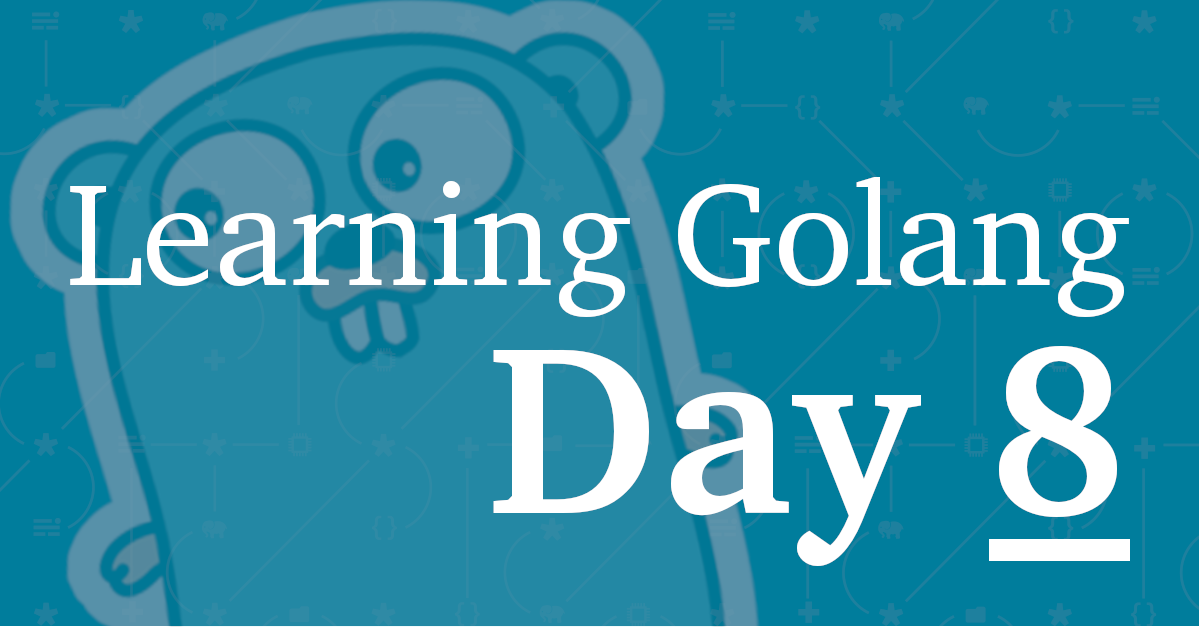# Learning Golang. Day 8Here we are on day 8. Today, I started learning about methods, pointer receivers, and interfaces.

It was a pretty good session as I have some prior experience with these aspects of Go, What’s more, I’ve been quite intrigued by them for ages because the way they’re implemented is notably different to most C-based OOP languages.

A method is just a function with a receiver argument.

Methods with pointer receivers can modify the value to which the receiver points

Take the following example, which I borrowed from the Go Tour.

`````` 1
2
3
4
5
6
7
8
9
10
11
12
13
14
15
16
17
18
19
package main

import (
"fmt"
"math"
)

type Vertex struct {
X, Y float64
}

func (v Vertex) Abs() float64 {
return math.Sqrt(v.X*v.X + v.Y*v.Y)
}

func main() {
v := Vertex{3, 4}
fmt.Println(v.Abs())
}
``````

In this example, the `Abs()` method is made available to the Vertex struct. In more explicit OOP languages, e.g, PHP, Python, or Java, you’d first have to declare a class, and then define the class as having the method, as in the PHP example below.

`````` 1
2
3
4
5
6
7
8
9
10
11
12
13
14
15
16
17
18
19
20
<?php

class Vertex
{
private float \$X;
private float \$Y;

public function __construct(float \$X, float \$Y)
{
\$this->X = \$X;
\$this->Y = \$Y;
}

public function Abs(): float {
return sqrt(\$this->X * \$this->X + \$this->Y * \$this->Y);
}
}

\$v = new Vertex(3, 4);
echo \$v->Abs();
``````

What’s happening in the example is quite clear. However, the Go example achieves the same result with fewer lines of code. As these two examples are quite short, the PHP example isn’t that much longer. In a production codebase, however, I suspect the reduced length for Go might become quite pronounced.

What’s also nice about the Go example here is that it doesn’t need an explicit constructor, nor does it need to declare the method’s visibility. Well, strictly-speaking, PHP doesn’t either as class methods are public by default. So I should be fair.

Another thing I like about the Go example is that, as the method receiver is a custom type – not a pointer to a custom type (a pointer receiver) – the method operates on a copy of the `Vertex` value. This is also similar to what I’m used to in PHP, such as where method variables are passed by value not by reference.

However, if the method receiver were a pointer receiver, in the following example, then the method would be able to modify the `Vertex` value, as in the example below, as then, in effect, `Vertex` would be passed by reference, such as when you prepend an ampersand to method arguments in PHP.

`````` 1
2
3
4
5
6
7
8
9
10
11
12
13
14
15
16
17
18
19
package main

import (
"fmt"
"math"
)

type Vertex struct {
X, Y float64
}

func (v *Vertex) Abs() float64 {
return math.Sqrt(v.X*v.X + v.Y*v.Y)
}

func main() {
v := &Vertex{3, 4}
fmt.Println(v.Abs())
}
``````

## Now, Let’s Talk About Go Interfaces

They’re yet another part of Go that I’m thinking I’ll really like, again, because the functionality is implicit, rather than (all too often) explicit.

An interface type is defined as a set of method signatures.

A type implements an interface if it implements the method. It doesn’t have to use a keyword such as `implements`, as PHP, Java, or other languages do.

Take the example below.

`````` 1
2
3
4
5
6
7
8
9
10
11
12
13
14
15
16
17
18
19
20
21
22
23
24
25
26
27
package main

import (
"fmt"
"math"
)

type Abser interface {
Abs() float64
}

type MyFloat float64

func (f MyFloat) Abs() float64 {
if f < 0 {
return float64(-f)
}
return float64(f)
}

type Vertex struct {
X, Y float64
}

func (v *Vertex) Abs() float64 {
return math.Sqrt(v.X*v.X + v.Y*v.Y)
}
``````

In this example, `Vertex` implements the `Abser` interface because it implements an `Abs()` method which has the same method signature as the one defined in the `Abser` interface. Short, sweet, and straight to the point. If you implemented it in Java, it’d look (something) like the following example.

`````` 1
2
3
4
5
6
7
8
9
10
11
12
13
14
15
16
17
18
19
20
21
22
23
24
public interface Abser {
float Abs();
}

public class Vertex implements Abser {
float X;
float Y;

public Vertex(float X, float Y) {
this.X = X;
this.Y = Y;
}

public float Abs() {
return (float) Math.sqrt(X * X + Y * Y);
}
}

public class Exercises {
public static void main(String[] args) {
Vertex v = new Vertex(3, 5);
System.out.println(v.Abs());
}
}
``````

However, I do acknowledge that as someone getting used to Go, if you’re coming from most other C-based languages, this approach may be quite confusing and lead to the code being hard to read. Also, sometimes being explicit can be a positive, as nothing’s left in any doubt.

I’m too new to this approach to really make a proper judgment call. But I will say that, at this stage, I like the brevity. Perhaps it’s because I’ve been living in Germany for nearly 10 years.

See you, tomorrow!

#learninggolang